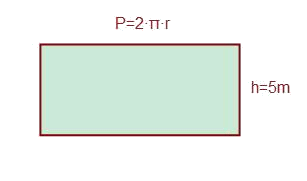# The cylinder: Surface area and volume

The cylinder is the surface with two circular bases joined by one side, as shown below:Calculate the area and volume of a cylinder with radius of the basis $$3 \ m$$ and height $$5 \ m$$.

The area of the basis will be:$$A_{basis}=2\cdot \pi \cdot r^2= 18\pi$$$For the area or the side it is interesting to open the side face in order to get the shape of a rectangle and to see that one side is the height of the cylinder and the other the perimeter of the basis.$$A_{lateral}=2 \pi \cdot r \cdot h =30 \pi \\A_{total}=48\pi$$$

The volume will have the following expression:$$V=\pi \cdot r^2 \cdot h \\ V=45 \pi \ m^3$$\$